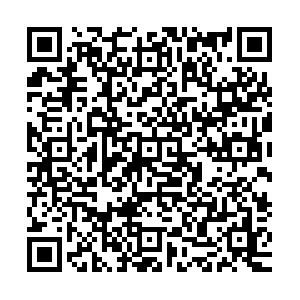# Double heavy tri-hadron bound state via delocalized π bond

• The number of exotic candidates that are beyond the conventional quark model has increased dramatically over recent decades. Some of these can be viewed as analogues of the deuteron. Similarly, the existence of the triton indicates that bound states formed by three hadrons could also exist. To illustrate this possibility, we study the DD*K and $B{B}^{* }\bar{K}$ systems using the Born-Oppenheimer approximation. To leading order, only one-pion exchange potentials are considered. This means that the three constituents share one virtual pion. This is similar to the role of the delocalized π bond for the formation of benzene in chemistry. After solving the Schrödinger equation, we find two three-body DD*K and $B{B}^{* }\bar{K}$ bound states with masses ${4317.92}_{-4.32}^{+3.66}\, {\rm{MeV}}$ and ${11013.65}_{-8.84}^{+8.49}\, {\rm{MeV}}$, respectively. The masses of their $D{\bar{D}}^{* }K$ and $B{\bar{B}}^{* }\bar{K}$ analogues are ${4317.92}_{-6.55}^{+6.13}\, {\rm{MeV}}$ and ${11013.65}_{-9.02}^{+8.68}\, {\rm{MeV}}$, respectively. From the experimental side, the $D{\bar{D}}^{* }K$ bound state could be found by analyzing the current world data on the $B\to J/\psi \pi \pi K$ process, by focusing on the J/ψπK channel.
••Get Citation
Li Ma, Qian Wang and Ulf-G. Meißner. Double heavy tri-hadron bound state via delocalized π bond[J]. Chinese Physics C, 2019, 43(1): 014102-1-014102-7. doi: 10.1088/1674-1137/43/1/014102
Li Ma, Qian Wang and Ulf-G. Meißner. Double heavy tri-hadron bound state via delocalized π bond[J]. Chinese Physics C, 2019, 43(1): 014102-1-014102-7.Milestone
Fund

Supported by the DFG (TR110) and the NSFC (11621131001) through funds provided to the Sino-German CRC 110 "Symmetries and the Emergence of Structure in QCD". The work of UGM was also supported by the Chinese Academy of Sciences (CAS) President's International Fellowship Initiative (PIFI) (2018DM0034) and by VolkswagenStiftung (93562)

Article Metric

Article Views(869)
Cited by(0)
Policy on re-use
To reuse of subscription content published by CPC, the users need to request permission from CPC, unless the content was published under an Open Access license which automatically permits that type of reuse.
###### 通讯作者: 陈斌, bchen63@163.com
• 1.

沈阳化工大学材料科学与工程学院 沈阳 110142

Title:
Email:

## Double heavy tri-hadron bound state via delocalized π bond

• 1. Helmholtz-Institut für Strahlen-und Kernphysik and Bethe Center for Theoretical Physics, Universität Bonn, D-53115 Bonn, Germany
• 2. Institut für Kernphysik, Institute for Advanced Simulation, and Jülich Center for Hadron Physics, Forschungszentrum Jülich, D-52425 Jülich, Germany
Fund Project:  Supported by the DFG (TR110) and the NSFC (11621131001) through funds provided to the Sino-German CRC 110 "Symmetries and the Emergence of Structure in QCD". The work of UGM was also supported by the Chinese Academy of Sciences (CAS) President's International Fellowship Initiative (PIFI) (2018DM0034) and by VolkswagenStiftung (93562)

Abstract: The number of exotic candidates that are beyond the conventional quark model has increased dramatically over recent decades. Some of these can be viewed as analogues of the deuteron. Similarly, the existence of the triton indicates that bound states formed by three hadrons could also exist. To illustrate this possibility, we study the DD*K and $B{B}^{* }\bar{K}$ systems using the Born-Oppenheimer approximation. To leading order, only one-pion exchange potentials are considered. This means that the three constituents share one virtual pion. This is similar to the role of the delocalized π bond for the formation of benzene in chemistry. After solving the Schrödinger equation, we find two three-body DD*K and $B{B}^{* }\bar{K}$ bound states with masses ${4317.92}_{-4.32}^{+3.66}\, {\rm{MeV}}$ and ${11013.65}_{-8.84}^{+8.49}\, {\rm{MeV}}$, respectively. The masses of their $D{\bar{D}}^{* }K$ and $B{\bar{B}}^{* }\bar{K}$ analogues are ${4317.92}_{-6.55}^{+6.13}\, {\rm{MeV}}$ and ${11013.65}_{-9.02}^{+8.68}\, {\rm{MeV}}$, respectively. From the experimental side, the $D{\bar{D}}^{* }K$ bound state could be found by analyzing the current world data on the $B\to J/\psi \pi \pi K$ process, by focusing on the J/ψπK channel.

### HTML关注分享DownLoad:  Full-Size Img  PowerPoint